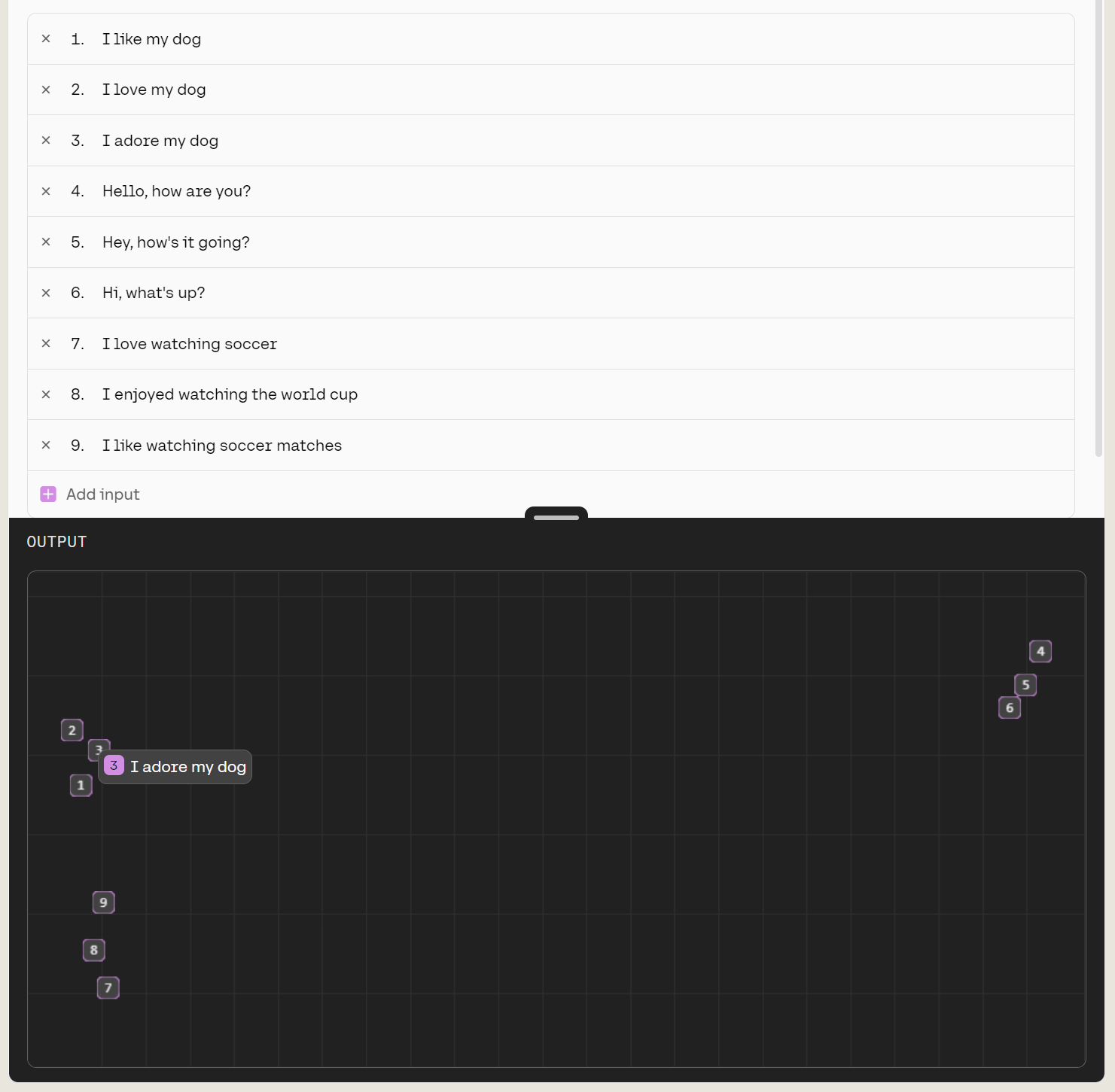# Embedding language in numbers: how LLMs represent meaningOne of the most powerful and fundamental transformations at the heart of how LLMs operate is the turning of words and sentences into numbers — numbers that are not arbitrary (like an index) but deeply, even mysteriously, meaningful.

The transformation process is called "embedding", and a simple way to think about it is to imagine a table comparing the words "car" to "dog". In this example, each word has a couple of attributes, which are scored in columns: on the "furry" attribute, "car" gets a score of zero and "dog" gets a score of 8, while on the "speed" attributed, "car" gets 7 and "dog" is scored as 3. To an LLM, the embedding for "car" is thus [0, 7], and the embedding for "dog" is [8, 3]. As mentioned above and as demonstrated here, these are not arbitrary numbers but reflections of real or perceived attributes.

Notice that [8, 3] is also a kind of coordinate, and if you plotted it on an x-y grid you'd have a dot marked "dog" at x=8 and y=3. Draw a line from the origin to that point and now you have a "dog" vector, described with the same two numbers. And if you do the same for "car" [0, 7], you'll get a vector for that word — and what's more, you'll be able to compare it geometrically to the "dog" vector to see how similar both vectors are to each other. For comparison, imagine a vector for "cat", which might be [7, 2] based on the attributes we're using. It's easy to visualize that the resulting "cat" vector will be much closer to the "dog" vector than the "car" vector is.

Sentences, too, have their embeddings. The screenshot from Cohere below shows how (in a simplified, flattened way so the process can be visualized) its LLM groups a list of sentences by similarity based on the meaning captured in the vectors that represent them.

Of course, real-life LLMs embed language structures at much vaster scales of complexity, inferring and using billions of attributes — some of which correspond to attributes a human might recognize (like "speed", above), while others correspond to important features the computer has recognized but which would be entirely baffling to a human.

Remarkable stuff.